Question

# 64. Benzene has a heat of vaporization of 30.72 kJ/mol and a normal boiling pressure is...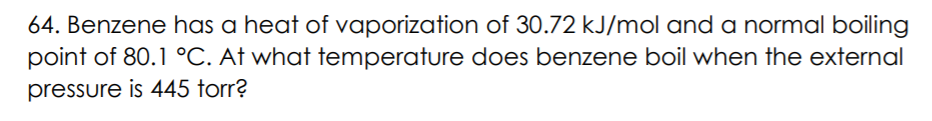64. Benzene has a heat of vaporization of 30.72 kJ/mol and a normal boiling pressure is 445 torr?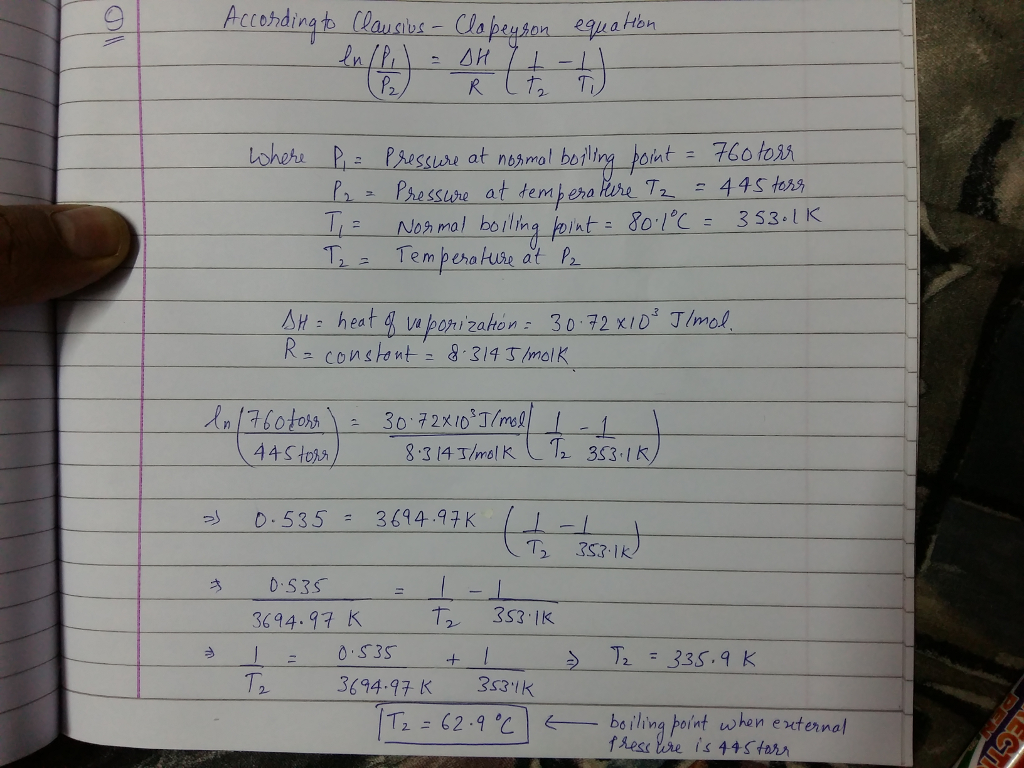#### Earn Coins

Coins can be redeemed for fabulous gifts.

Similar Homework Help Questions
• ### Benzene has a heat of vaporization of 30.72 kJ/mol and a normal boiling point of 80.1...

Benzene has a heat of vaporization of 30.72 kJ/mol and a normal boiling point of 80.1 °C. At what temperature does benzene boil when the external pressure is 445 torr?

• ### benzene has a heat of vaporization of 30.72kj/mol and a normal boiling point of 80.1c

benzene has a heat of vaporization of 30.72kj/mol and a normal boiling point of 80.1c. at what temperature does benzene boil when the external pressure is 445 torr

• ### Benzene has a heat of vaporization of 30.72kj/mol and a normal boiling point of 80.1 C

Benzene has a heat of vaporization of 30.72kj/mol and a normal boiling point of 80.1 C . At what temperature does benzene boil when the external pressure is 445 ? This is how I am solving this problem, but when I enter the answer it says im wrong. Can someone help with the math. Thanksln(445/760)= (30720/8.314)*(1/80 - 1/T2)

• ### The heat of vaporization of benzene c6h6 , is 30.8 kJ/mol at its boiling point of...

The heat of vaporization of benzene c6h6 , is 30.8 kJ/mol at its boiling point of 80.1 degrees Celsius. How much energy in the form of heat is required to vaporize 128 g benzene at its boiling point?

• ### 20.Ethanol has a heat of vaporization of 38.56 kJ/mol and a normal boiling point of 78.4...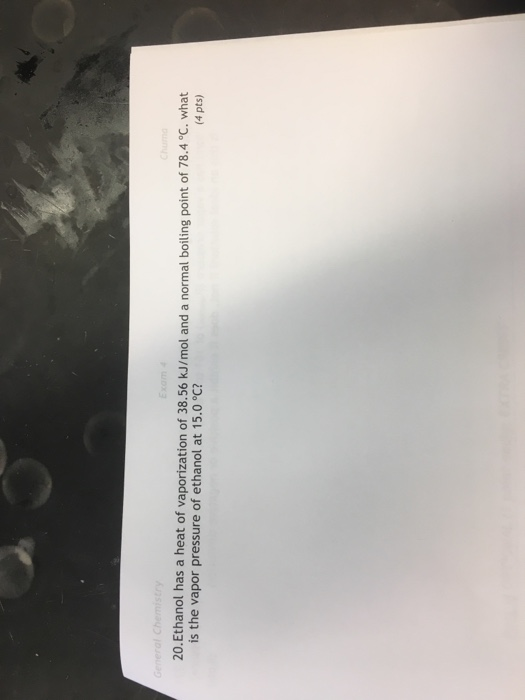20.Ethanol has a heat of vaporization of 38.56 kJ/mol and a normal boiling point of 78.4 °C. what is the vapor pressure of ethanol at 15.0 °C? 4 pts)

• ### Part A Which substance has the highest boiling point? ОСО CH3OH N2 Submit Request Answer Benzene...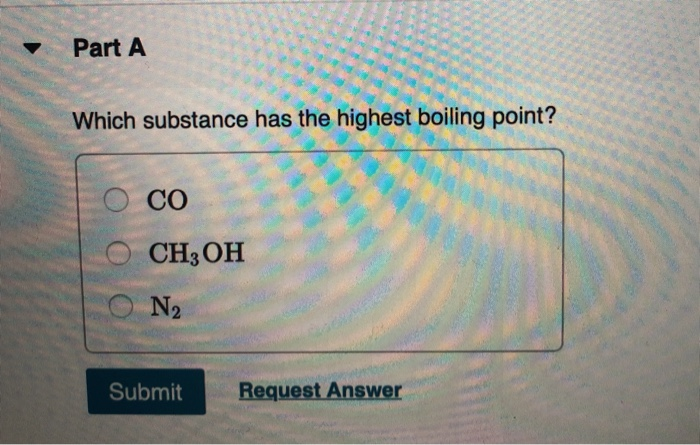Part A Which substance has the highest boiling point? ОСО CH3OH N2 Submit Request Answer Benzene has a heat of vaporization of 30.72 kJ/mol and a normal boiling point of 80.1 °C. You may want to reference (Pages 503 - 512) section 11.5 while completing this problem. At what temperature does benzene boil when the external pressure is 405 torr ? Express your answer in degrees Celsius using two significant figures. IVO ΑΣΦ ? T = °C Submit Request Answer

• ### 1. The normal boiling point of benzene (i.e., at 1 atm) is 80.09 °C. The molar...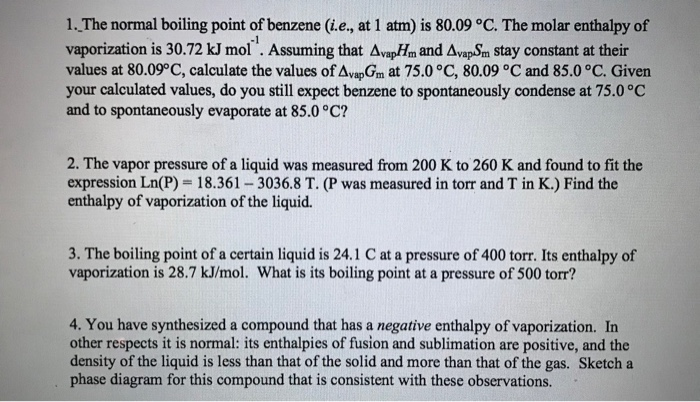1. The normal boiling point of benzene (i.e., at 1 atm) is 80.09 °C. The molar enthalpy of vaporization is 30.72 kJ mol . Assuming that AvapHm and AvapSm stay constant at their values at 80.09°C, calculate the values of AvapGm at 75.0 °C, 80.09 °C and 85.0 °C. Given your calculated values, do you still expect benzene to spontaneously condense at 75.0 °C and to spontaneously evaporate at 85.0°C? -1 2. The vapor pressure of a liquid was measured...

• ### Given the heat of vaporization of benzene as 30.8 kJ/mol and the vapor pressure = 92...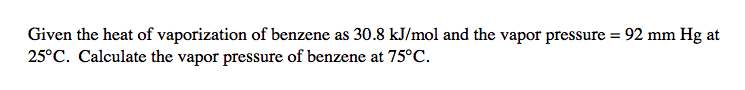Given the heat of vaporization of benzene as 30.8 kJ/mol and the vapor pressure = 92 mm Hg at 25°C. Calculate the vapor pressure of benzene at 75°C.

• ### I keep getting the wrong answer. Can anyone solve this temperature problem

Benzene has a heat of vaporization of 30.72 kJ/mol and a normal boiling point of 80.1 degrees C. At what temperature does benzene boil when the external pressure is435 torr ?

• ### The molar enthalpy of vaporization of carbon disulfide is 26.74 kJ/mol, and its normal boiling point...

The molar enthalpy of vaporization of carbon disulfide is 26.74 kJ/mol, and its normal boiling point is 46°C. What is the vapor pressure of CS2 at 0°C? a)447 torr b)4160 torr c)313 torr d)139 torr e)5.47 torr